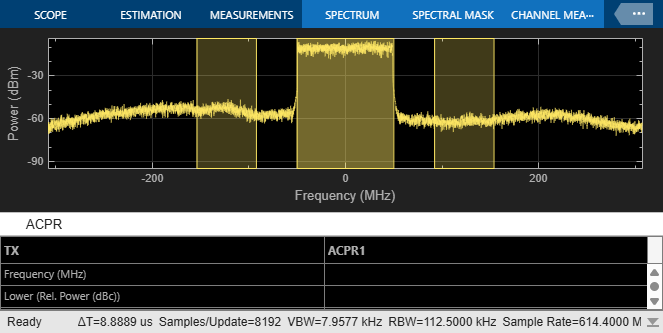# Speed Up PA and DPD simulation

This example shows how to simulate a power amplifier (PA) within a wireless communications system and how to apply digital predistortion (DPD) to improve the transmitter linearity. The simulation speed of the model is increased by using the Idealized Baseband library and hence increasing the abstraction level of the model.

### Idealized Baseband Library Model for Two Tones Input Signal

The first model of the RF system uses blocks from the RF Blockset™ Idealized Baseband library. With this library an equivalent baseband modeling approach is adopted. Complex modulated signals represent the transmitted information, assumed to be centered around an implicit carrier frequency.

The blocks from the Idealized Baseband library do not model out-of-band behavior or spurious harmonics generated by nonlinear effects or interfering signals. Additionally, blocks from the Idealized Baseband library do not model impedance mismatches and assume that all blocks are perfectly matched to 50 ohms. As a result, RF models built with the Idealized Baseband library simulate quickly.

The first testbench used for PA and DPD simulation generates two tones to test the PA with or without DPD. In this case, test the model with a narrowband excitation. You can toggle the manual switch in the simulation and verify that the overall IP3 performance of the system is improved.

```Elapsed time is 3.537725 seconds. ```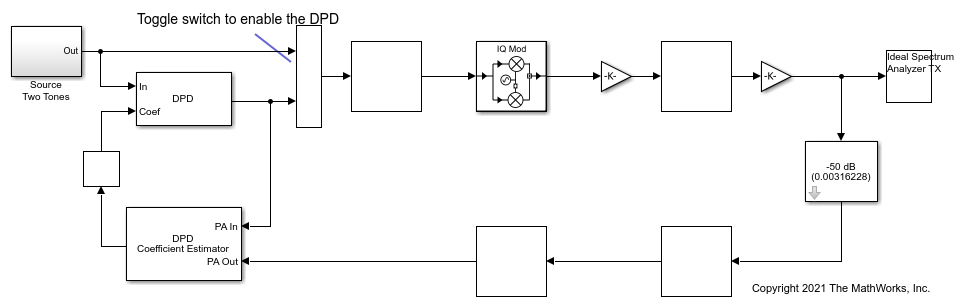Enable the DPD and verify the improvement on linearity.

```Elapsed time is 1.877610 seconds. ```To validate the fidelity of the model, compare it with an equivalent implementation built using blocks from the Circuit Envelope library. In this case, multicarrier effects, second order nonlinearity, and impedance mismatches are taken into account.

```Elapsed time is 52.307800 seconds. ```Enable the DPD and verify the improvement on linearity.

```Elapsed time is 50.682618 seconds. ```Results obtained with the two models are very similar, but the simulation speed of the idealized baseband model is much faster.

### Idealized Baseband Library Model for OFDM Input Signal

Test the Idealized Baseband library model using a modulated OFDM input signal. In this case, the baseband signal has a bandwidth of 100 MHz. The simulation bandwidth of the model has been increased by a factor of five to improve the capture of in-band effects (spectral regrowth) resulting from nonlinearities. EVM is computed using the constellation diagram and ACLR using the spectrum analyzer scope.

In this second testbench, test the RF system and its linearization with a wideband signal. Note that the PA coefficients now represent a different device compared to the one used in the first testbench and that the PA has been characterized for wideband operation.

```Elapsed time is 2.042193 seconds. ```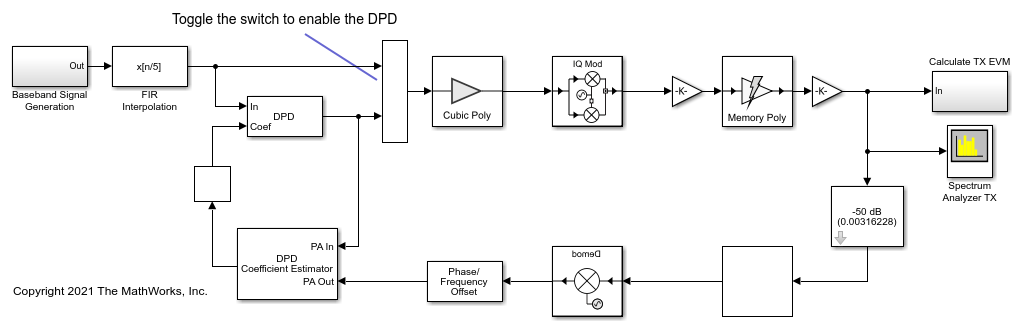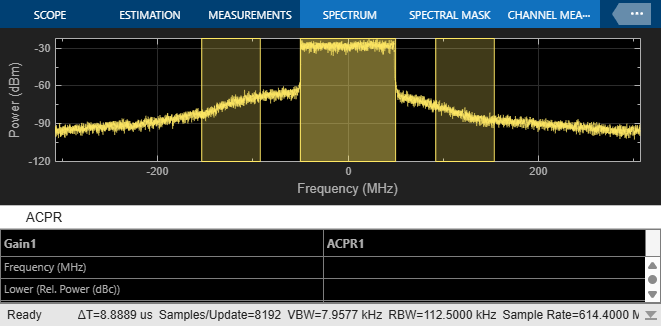Enable the DPD and verify the improvement on linearity.

```Elapsed time is 1.733932 seconds. ```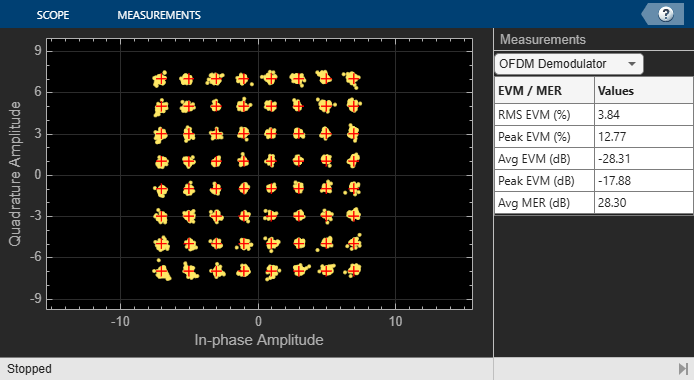Compare the results obtained with an equivalent model built using blocks from the Circuit Envelope Library. In this case the effects of the antenna loading on the coupler and PA are taken into account. These effects lead to additional differences when compared to the model built using blocks from the Idealized Baseband Library.

```Elapsed time is 169.742750 seconds. ```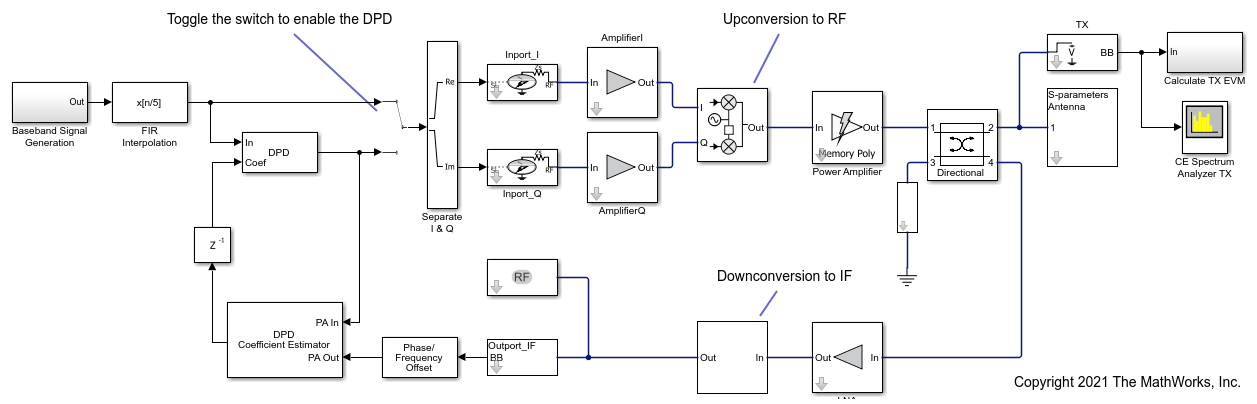Enable the DPD and verify the improvement on linearity.

```Elapsed time is 165.454819 seconds. ```